Statistics Discussion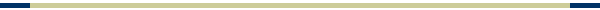1       Introduction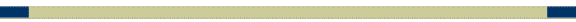# 9      Theoretical Distributions Including the Normal Distribution

## 9.6   The Normal Distribution

### 9.6.6   Finding the Score that Separates the Population into Two Proportions

#### 9.6.6.4                 Procedure

##### 9.6.6.4.2    The product of step # 1 above is used to calculate the z score for the above equation. To find the z score use the use tables in a stats textbook in the table for areas under the normal curve between the mean and z. Look up the difference in the previous step or its closest approximation and find the z score associated to plug into the equation above. You can also use the web reference below to find the z score
###### 9.6.6.4.2.1   Web Reference

9.6.6.4.2.1.1     The Web reference below 2nd applet gives you the z score to be used in the equation above. Pug in a mean of 0 and SD (Standard Deviation) of 1, put in the percentage in decimals (eg .10=10%, .20=20%) into the shaded area box, and click the above button to obtain the z score you can use in the above equation.

9.6.6.4.2.1.2     http://davidmlane.com/hyperstat/z_table.html

# 10      Samples and Sampling Distributions

## 10.4 The Sampling Distribution of the Mean

### 10.4.1            Introduction

#### 10.4.1.1             Empirical Sampling Distribution of the Mean

##### 10.4.1.1.2                  Illustration
###### 10.4.1.1.2.2                Empirical sampling Distribution of the Means (Frequency Distribution of sample means)

10.4.1.1.2.2.1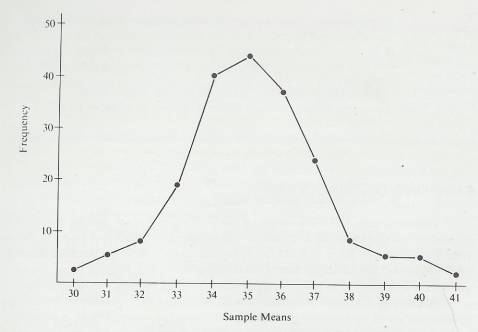##### 10.4.1.1.11              Illustration Theoretical Sampling Distribution of the Mean, N=10
###### 10.4.1.1.11.1            Population

10.4.1.1.11.1.1          Mean=35.375

10.4.1.1.11.1.2          Standard Deviation=6.304

###### 10.4.1.1.11.2            Sampling Distribution of the Mean for N=10

10.4.1.1.11.2.1          Mean=35.375

10.4.1.1.11.2.2          Standard Deviation=6.304/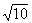=1.993

###### 10.4.1.1.11.3            Illustration

10.4.1.1.11.3.1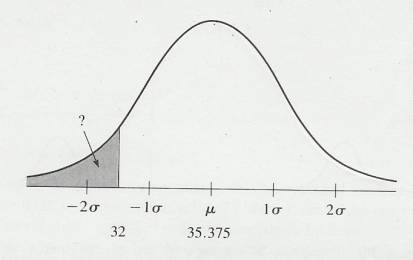# 11      Differences between Means

## 11.6 How to Construct A Sampling Distribution of Differences Between Means

### 11.6.2            General Formula

#### 11.6.2.9             Discussion

##### 11.6.2.9.3                  Finding the z score associated with the probabilities
###### 11.6.2.9.3.1                There are at least two ways of determining the z score associated with the above probabilities

11.6.2.9.3.1.1               Look up the z score in a table in the back of a stats text book. To do this you will need to subtract the probabilities above from .5000 to find the correct z score which will give you the proportions

11.6.2.9.3.1.1.1                  .25 .375 .475 .495 .4995

11.6.2.9.3.1.1.2                  Look these up in a table in the back of a sts text book to find the z scores listed below plug oin the following probability figures .25 .125 .025 .005 .0005

11.6.2.9.3.1.2               Using the web reference below

11.6.2.9.3.1.2.1                  Plug in the following probabilities  .25 .125 .025 .005 .0005 into the shaded area of the 3rd applet and click the above or below button

11.6.2.9.3.1.2.2                  Web Reference

11.6.2.9.3.1.2.2.1                http://davidmlane.com/hyperstat/z_table.html

11.6.2.9.3.1.2.3                  The following z scores are associated

11.6.2.9.3.1.2.3.1                .67 1.15 1.96 2.58 3.30

#### 11.6.2.10        Simple z score method (z Score For Observed Mean Difference ) (No Charting)

##### 11.6.2.10.1              Procedure
###### 11.6.2.10.1.1            Simply determine the z Score For Observed Mean Difference go to the 2nd applet from the web reference below, and plug in the z score to determine the proportion above the z score to determine proportion occurring by chance.

11.6.2.10.1.1.1.1             Web Reference

11.6.2.10.1.1.1.1.1            http://davidmlane.com/hyperstat/z_table.html

## 11.10                   How To Reject the Null Hypothesis

### 11.10.2      In order to get this discussion out of the realm of the hypothetical and into the realm of the practical, consider the following problem. Supposing you want to select a research project which seeks to reject H0. You decide to try to show that widgets are different from controls. Accept for a moment the idea that widgets are different-that H0 should be rejected. What are the factors that determine whether you will conclude from your experiment that widgets are different?

#### 11.10.2.2        The Standard Error of a Difference

##### 11.10.2.2.2              You can see that as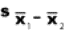gets smaller, z gets larger, and you are more likely to reject H0. This is true, of course, only if widgets are really different from controls. Here are two ways you can reduce the size of.
###### 11.10.2.2.2.1            Sample Size

11.10.2.2.2.1.1          The larger the sample, the smaller the standard error of the difference. (See Illustration) This illustration shows that the larger the sample size, the smaller the standard error of the mean. The same relationship is true for the standard error of a difference.

11.10.2.2.2.1.2          Some Texts  show you how to calculate the sample size required to reject H0. In order to do this calculation, you must make assumptions about the size of the actual difference. Many times, the size of the sample is dictated by practical consideration-time, money, or the availability of widgets.

###### 11.10.2.2.2.2            Sample variability

11.10.2.2.2.2.1          Reducing the variability in the sample will produce a smaller. You can reduce variability by using reliable measuring instruments, recording data correctly, and, in short, reducing the “noise” or random error in your experiment.

# 12      The t Distribution and the t-Test 

## 12.5 Using the t Distribution for Independent Samples

### 12.5.16      Formulas and Procedure

#### 12.5.16.1        Standard error of the difference between means

##### 12.5.16.1.1N1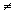N2
###### 12.5.16.1.1.1Formula

12.5.16.1.1.1.1          Raw Score Formulas

12.5.16.1.1.1.1.1

12.5.16.1.1.1.2          Deviation Score Formulas

12.5.16.1.1.1.2.1

###### 12.5.16.1.1.2Procedure

12.5.16.1.1.2.1

###### 12.5.16.1.1.3Variables Defined

12.5.16.1.1.3.1

##### 12.5.16.1.2N1=N2
###### 12.5.16.1.2.1Formula

12.5.16.1.2.1.1          Raw Score Formulas

12.5.16.1.2.1.1.1

12.5.16.1.2.1.2          Deviation Score Formulas

12.5.16.1.2.1.2.1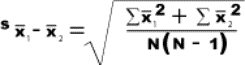###### 12.5.16.1.2.2Variables Defined

12.5.16.1.2.2.1=Standard error of the difference between means

###### 12.5.16.1.2.3Procedure

12.5.16.1.2.3.1

## 12.6 Using the t Distribution for Correlated Samples (Some texts use the term dependent samples instead of correlated sample)s

### 12.6.5            Calculating a t Value for Correlated Samples

#### 12.6.5.19        Illustration Formulas

##### 12.6.5.19.2Variables Defined
###### 12.6.5.19.2.1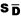=standard error of the difference between correlated means (direct-difference method)

12.6.5.19.2.1.1.1=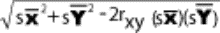##### 12.6.5.19.5              Procedure
###### 12.6.5.19.5.1            s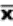or s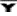=Standard Error of Mean

12.6.5.19.5.1.1          s12.6.5.19.5.1.1.1             Determine the standard deviation of X scores

12.6.5.19.5.1.1.2             Determine the square root of the total number of scores

12.6.5.19.5.1.1.3             Divide the product of step #1 (standard deviation of X scores) by the product of step #2 (square root of the number of X scores)

12.6.5.19.5.1.2          s12.6.5.19.5.1.2.1             Determine the standard deviation of Y scores

12.6.5.19.5.1.2.2             Determine the square root of the total number of scores

12.6.5.19.5.1.2.3             Divide the product of step #1 (standard deviation of Y scores) by the product of step #2 (square root of the number of Y scores)

12.6.5.19.5.1.312.6.5.19.5.1.3.1             Square s(multiply it by itself)

12.6.5.19.5.1.3.2             Square s(multiply it by itself)

12.6.5.19.5.1.3.3             Add Squared sto Squared s12.6.5.19.5.1.3.4             Determine the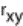(Correlation between X & Y)

12.6.5.19.5.1.3.5             Multiply theby 2

12.6.5.19.5.1.3.6             Multiply sby s12.6.5.19.5.1.3.7             Multiply the product of step #6 (sXs s) by the product of step #5 (Xs 2)

12.6.5.19.5.1.3.8             Subtract the product of step #7 ((Xs 2)  Xs (sXs s)) from the product of step #3 (Squared s+ Squared s)

12.6.5.19.5.1.3.9             Obtain the square root of step #8 to obtain thescore

###### 12.6.5.19.5.2(t) value

12.6.5.19.5.2.1

#### 12.6.5.20        Computation Formula (Direct-Difference Method)

##### 12.6.5.20.2Variables Defined
###### 12.6.5.20.2.1= standard error of the difference between correlated means (direct-difference method)

12.6.5.20.2.1.1=###### 12.6.5.20.2.2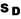=Standard deviation of the distribution of differences between correlated scores (direct-difference method)

12.6.5.20.2.2.1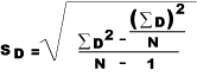##### 12.6.5.20.3Procedure
###### 12.6.5.20.3.1() Standard deviation of the distribution of differences between correlated scores (direct-difference method)

12.6.5.20.3.1.1          Create a column with the difference between the means. That is find the difference between each pretest and posttest  score (minus the posttest from the pretest) and put that number in a column

12.6.5.20.3.1.2          Create a column with the squared differences between the means. That is multiply the difference between the means by itself

12.6.5.20.3.1.3          Sum the column of squared differences (the column created in step 2)

12.6.5.20.3.1.4          Sum the column of differences (step1) and square the sum (multiply it by itself). Then divide this product by the number of score pairs.

12.6.5.20.3.1.5          Minus the product of the previous step (step 4) from the sum of the squared differences (step 3)

12.6.5.20.3.1.6          Take the number of score pairs and minus 1 from that number

12.6.5.20.3.1.7          Divide the product of step 5 by the product of step 6 to determine the () score

###### 12.6.5.20.3.2(t) Score

12.6.5.20.3.2.1          Find the difference betweenand12.6.5.20.3.2.2          Obtain the square root of the number of score pairs

12.6.5.20.3.2.3          Divideby the product of step 2 to obtain the t score

12.6.5.20.3.2.4

## 12.7 Using the t Distribution to Establish a Confidence Interval about a Mean Difference

### 12.7.3            Confidence Intervals for Correlated Samples

#### 12.7.3.7             Formulas

##### 12.7.3.7.3                  Variables Defined
###### 12.7.3.7.3.1= standard error of the difference between correlated means (direct-difference method)

12.7.3.7.3.1.1=###### 12.7.3.7.3.6                df=the degrees of freedom for this equation is N-1

12.7.3.7.3.6.1               Example

12.7.3.7.3.6.1.1                  http://www.psychstat.smsu.edu/introbook/tdist.htm

##### 12.7.3.7.4                  Procedure
###### 12.7.3.7.4.1                Upper Confidence Interval Calculation

12.7.3.7.4.1.1               Subtract the Mean of Y scores from the Mean of X scores

12.7.3.7.4.1.2               Multiplyby the t score found in the table. Look across from the degrees of freedom (N-1) and under the alpha level .05. .02, .001 ect

12.7.3.7.4.1.3               Add the product of step #1 to the product of step #2 for the upper limit confidence interval

###### 12.7.3.7.4.2                Lower Confidence Interval Calculation

12.7.3.7.4.2.1               Subtract the Mean of Y scores from the Mean of X scores

12.7.3.7.4.2.2               Multiplyby the t score found in the table. Look across from the degrees of freedom (N-1) and under the alpha level .05. .02, .001 ect

12.7.3.7.4.2.3               Subtract the product of step #1 to the product of step #2 for the lower limit confidence interval